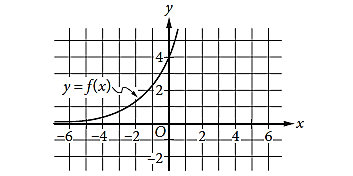Maths-
General
Easy

Question

#The graph of y=f(x)   is shown in the xy-plane. What is the value of  f(0) ?

## 0234Hint:

## The correct answer is: 4

### Explanation: We have given the graph of a function f(x). We have to find the value of f(0). .Step 1 of 1:We have given the graph of a function f(x).Now for f(0) , we know that x=0 it means we have to find the point where the graph cuts y-axis.From the graph, we can clearly say that the value of the function f(x) at  x=0 is 4.Therefore, the value of f(0)  will be 4.Hence, Option D is correct.#### With Turito Foundation.#### Get an Expert Advice From Turito.